Courses

# Test: Spectroscopy- 1

## 35 Questions MCQ Test Organic Chemistry | Test: Spectroscopy- 1

Description
This mock test of Test: Spectroscopy- 1 for Chemistry helps you for every Chemistry entrance exam. This contains 35 Multiple Choice Questions for Chemistry Test: Spectroscopy- 1 (mcq) to study with solutions a complete question bank. The solved questions answers in this Test: Spectroscopy- 1 quiz give you a good mix of easy questions and tough questions. Chemistry students definitely take this Test: Spectroscopy- 1 exercise for a better result in the exam. You can find other Test: Spectroscopy- 1 extra questions, long questions & short questions for Chemistry on EduRev as well by searching above.
QUESTION: 1

Solution:
QUESTION: 2

Solution:
QUESTION: 3

### In the IR spectrum, carbonyl absorption band for the following compound appears at: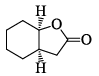Solution:
QUESTION: 4

In the IR spectrum of p-nitrophenyl acetate, the carbonyl absorption band appears at:

Solution:
QUESTION: 5

A triatomic molecule of the type AB2 shows two IR absorption lines and one IR-Raman line. The structure of the molecule is:

Solution:
QUESTION: 6

In the IR spectrum, the absorption band due to carbonyl group in phenyl acetate appears at:

Solution:
QUESTION: 7

In the IR spectrum of p-nitrophenyl, acetate, the carbonyl absorption band appears at:

Solution:
QUESTION: 8

A compound with molecule formula C4H6O2 shows band at 1170 cm–1 in IR spectrum and peaks at 178, 68, 28 and 22 ppm in 13C NMR spectrum. The correct structure of the compound is:

Solution:
QUESTION: 9

The compound that exhibits sharp bands at 3300 and 2150 cm–1 in the IR spectrum is:

Solution:
QUESTION: 10

Correctly matched structure and carbonyl stretching frequency set is: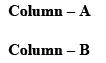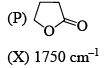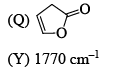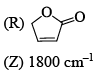Solution:
QUESTION: 11

Among the following, the compound that displays an IR band at 2150 cm–1 is:

Solution:
QUESTION: 12

The compound which shows IR frequencies at both 3314 and 2126 cm–1 is:

Solution:
QUESTION: 13

Match the compounds in List – I with the stretching frequencies (cm–1) of the principal funct ional groups given in List – II.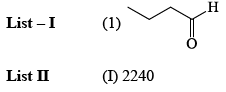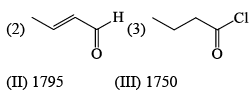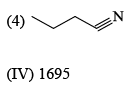Solution:
QUESTION: 14

The IR stretching frequencies (cm–1) for the compound X are as follows: 3300-3500 (s, br); 3000 (m); 2225 (s); 1680(s). The correct assignment of the absorption bands is: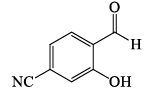Solution:
QUESTION: 15

Among the compounds given in the option (a) to (d), the one that exhibits a sharp band at around 3300 cm–1 in the IR spectrum is:

Solution:
QUESTION: 16

The correct order of IR stretching frequency of the C=C in the following olefins is: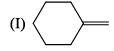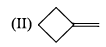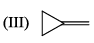Solution:
QUESTION: 17

Arrange the chacacteristic timescales of the following set of dynamical spectroscopic techniques in decreasing order of time (longest to shortest); NMR (Nuclear Magnetic Resonance), ESR (Electron Spin Resonance), Fluorescence, Raman and Absorption.

Solution:
QUESTION: 18

What are the limits of detection of the following common analyt ical methods used with capillar y separations: fluorescence, mass spectrometry, UV-Vis absorbance, and NMR, respectively, in mol:

Solution:
QUESTION: 19

Of the molecules CH4, CO2, benzene and H2, the ones that will absorb infrared radiat ion are:

Solution:
QUESTION: 20

IR stretching frequencies of carbonyl groups in aldehydes and acid chlorides in cm–1 are:

Solution:
QUESTION: 21

The bond that gives the most intense band in the infrared spectrum for its stretching vibrat ions is:

Solution:
QUESTION: 22

An examinations of saturated hydrocarbons containing methyl group show asymmetrical (Vas) and symmetrical (Vs) stretching modes in the region of:

Solution:
QUESTION: 23

For each set of compounds below, choose the one in which the indicated hydrogen is farthest upfield in a proton NMR spectrum: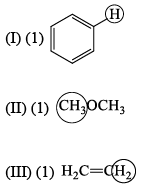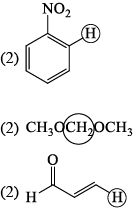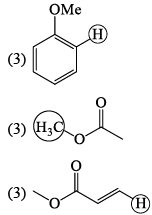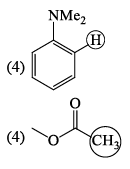Solution:

In NMR spectroscopy upfield means the shielded protons. Hence the farthest upfield are the ones that are most effectively shielded from NMR spectroscopy rays.

According to the above discussion A is correct.

QUESTION: 24

The molecule with the smallest rotational constant (in the microwave spectrum) among the following is:

Solution:
QUESTION: 25

N2 does not show pure vibrat ional spectral because:

Solution:
QUESTION: 26

Which of the following molecules has the lowest vibrational stretching frequency:

Solution:
QUESTION: 27

A radiat ion which has an energy of N 50 kJ mol–1 falls in the following region of the electromagnetic spectrum:

Solution:
QUESTION: 28

The rotational constant (B) of H35Cl, H37Cl and D35Cl follow the order:

Solution:
QUESTION: 29

The spectroscopic data for an organic compound with molecular formula C10H12O2 are given below. IR band around 1750 cm–1. 1H NMR d 7.3 (m, 5H), 5.85 (q, 1H, J = 7.2 Hz), 2.05 (s, 3H), 1.5 (d, 3H, J = 7.2 Hz) ppm. The compound is:

Solution:
QUESTION: 30

The correct order of the 1H NMR chemical shift values (β) for the indicated hydrogens (in bold) in the following compounds is: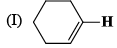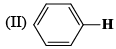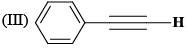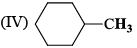Solution:
QUESTION: 31

The correct order of 1H NMR chemical shift (β) values for the labeled methyl groups in the following compound is: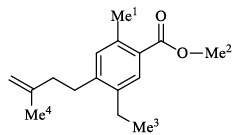Solution:
QUESTION: 32

A C5H12O2 compound has strong infrared absorption at 3300 to 3400cm-1. The 1H NMR spectrum has three singlets at β 0.9, β 3.45 and β 3.2 ppm with relative areas 3:2:1. Addition of D2O to the sample eliminates the lower field signal. The 13C NMR spectrum shows three signals all at higher field than β 100 ppm. Which of the following compounds best fits this data:

Solution:
QUESTION: 33

A compound of formula C5H12 gives one signal in the 1H NMR and two signals in the 13C NMR spectra. The compound is:

Solution:
QUESTION: 34

The most appropriate spectroscopy for the identification of a nitrile group is:

Solution:
QUESTION: 35

Compound I gives a strong infrared absorption at 1730 cm-1. 1H NMR spectrum indicates that it has two types of hydrogen atoms; one H atom appearing as signlet at δ = 9.7 ppm and 9H atoms appearing as a singlet at δ = 1.2 ppm. The structure of I is:

Solution: